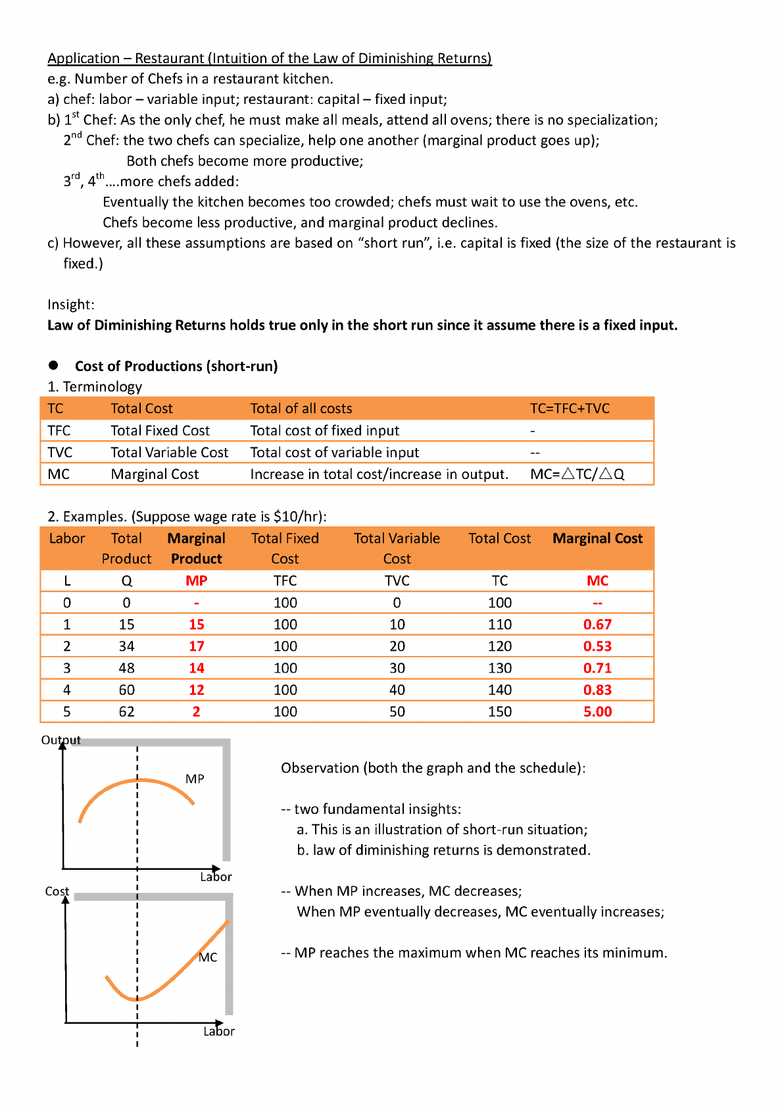# ECO101H1 Lecture Notes - Diminishing Returns, Marginal Cost, Marginal Product

51 views6 pages
School
Department
Course
ProfessorTopic 7 t Production & Cost Schedule
(Week seven Oct 25th-Nov 1st)
Outline:
1. Production Function;
2. Product Schedule (Short Run)
-- Definition;
-- Law of Diminishing Returns;
3. Cost of Productions (Short Run)
-- Terminology;
-- Examples;
-- Properties of firms[ cost curves;
4. Average Costs
-- Terminology
-- Examples;
-- why curves are shaped that way and their intercepts;
4. Long-Run Average Cost Curve;
5. Opportunity Cost and the Measurement of Economic Profit
z Production Function: relationship between output and the quantity of input.
Short-run: one input (capital) is fixed; while on input (labor) can vary;
Long-run: all inputs (capital, labor, etc.) can vary.
-- e.g. General Motors;
Short-run: GM can vary the amount of labor (overtime, lay-offs), but cannot vary the number of
plants (selling land);
Long-run: GM can vary both number of plants and amount of labor;
z Product Schedules (short-run)
1. Terminology
TP
Total Product
Total output, given the labor input
MP
Marginal Product
Increase in total output divided by increase in labor input;
AP
Average Product
Total product divided by labor input.
2. Law of Diminishing Returns
The marginal product of a variable input, in the presence of a fixed input, eventually diminishes.
e.g. Numerical Example
Labor
TP
MP
AP
0
0
1
4
4
4
2
10
6
5
3
13
3
4.33
4
15
2
3.75
5
16
1
3.20
Observation:
a. This illustration strictly accords with the law of
diminishing returns
(as labor increases, MP eventually decreases.).
b. If MP is above AP, AP is rising; if MP is less than AP, AP is
falling.
(this is merely mathematical observation, no economic
significance is involved. )
www.notesolution.com
Unlock document

This preview shows pages 1-2 of the document.
Unlock all 6 pages and 3 million more documents.www.notesolution.com
Unlock document

This preview shows pages 1-2 of the document.
Unlock all 6 pages and 3 million more documents.

## Document Summary

Topic 7 j production & cost schedule (week seven oct 25th-nov 1st) Outline: production function, product schedule (short run) - law of diminishing returns: cost of productions (short run) - properties of firms cost curves: average costs. - why curves are shaped that way and their intercepts: long-run average cost curve, opportunity cost and the measurement of economic profit. production function: relationship between output and the quantity of input. Short-run: one input (capital) is fixed; while on input (labor) can vary; Long-run: all inputs (capital, labor, etc. ) can vary. Short-run: gm can vary the amount of labor (overtime, lay-offs), but cannot vary the number of plants (selling land); Long-run: gm can vary both number of plants and amount of labor; Increase in total output divided by increase in labor input; Total product divided by labor input: law of diminishing returns. The marginal product of a variable input, in the presence of a fixed input, eventually diminishes. e. g. numerical example.

## Get access

\$8 USD/m\$10 USD/m
Billed \$96 USD annuallyHomework Help
Study Guides
Textbook Solutions
Class Notes
Textbook Notes
Booster Class